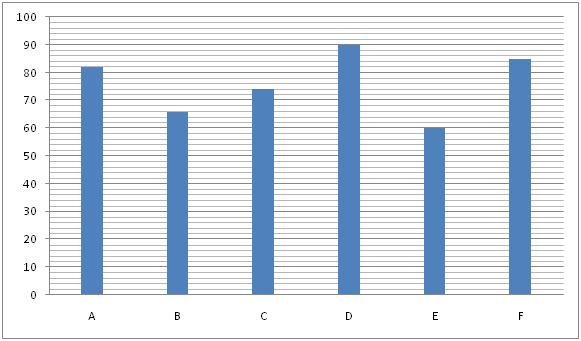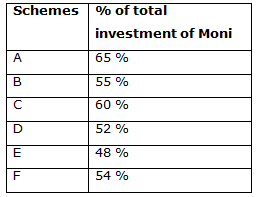# IBPS PO Mains 2018 – Quantitative Aptitude Questions Day-16

Dear Readers, Bank Exam Race for the Year 2018 is already started, To enrich your preparation here we have providing new series of Practice Questions on Quantitative Aptitude – Section. Candidates those who are preparing for IBPS PO Mains 2018 Exams can practice these questions daily and make your preparation effective.

[WpProQuiz 4155]

1) 5 years ago, the age of the father is 3 times the age of his son. 7 years hence, the age of the father and his son is in the ratio of 9: 4. The average Present age of the father, mother, son and daughter is 40. The difference between the age of the mother and her daughter is 34. Then find the present age of the daughter?

a) 18 years

b) 20 years

c) 16 years

d) 22 years

e) None of these

2) P, Q and R started a business by investing in the ratio of 6: 8: 7. After 5 months, P withdraws Rs. 4000 and after another 2 months, R invested Rs. 2000 more. If the share of P, Q and R is in the ratio of 130: 192: 173, then find the initial investment of R?

a) Rs. 21000

b) Rs. 42000

c) Rs. 28000

d) Rs. 35000

e) None of these

3) The shopkeeper gives 20 % discount on the marked price for selling an article. If the cost price of the article is increased by 10 %, then the profit becomes half while comparing with the original profit. The marked price of the article is Rs. 600. Find the cost price of the article?

a) Rs. 440

b) Rs. 380

c) Rs. 400

d) Rs. 360

e) None of these

4) Three pipes A, B and C can fill a tank in 12 hours. After working at it together for 4 hours, C is closed and A and B can fill the remaining part in 12 hours. How much time taken by C to fill the tank alone?

a) 30 hours

b) 36 hours

c) 40 hours

d) 42 hours

e) None of these

5) 18 men can complete a piece of work in 10 days. After 4 days from the start of the work, some men left. If the remaining work was completed by the remaining men in 9 days, then how many men left after 4 days from the start of the work?

a) 5 men

b) 4 men

c) 3 men

d) 6 men

e) None of these

Directions (Q. 6 – 10) Study the following information carefully and answer the given questions.

The following bar graph 1 shows the total investment (In thousands) of Moni and Priya in 6 different schemes (A, B, C, D, E and F)The following table shows the percentage of total investment of Moni in 6 different schemes (A, B, C, D, E and F) out of the total investment of Moni and Priya in the same schemes.6) If scheme C offers simple interest at a certain rate of interest and the difference between the interest earned by Moni and Priya from scheme C after 3 years is Rs. 3552, then find the rate of interest per annum?

a) 10 %

b) 12 %

c) 15 %

d) 8 %

e) None of these

7) Scheme A offers compound interest compounded annually at 15 % per annum. Find the sum of the interests earned by Moni and Priya after 2 years in Scheme A?

a) Rs. 29560

b) Rs. 26445

c) Rs. 31252

d) Rs. 27517

e) None of these

8) The amount invested by Moni in Scheme E is equal to the amount invested by her in Scheme P. The rate of interest for both the schemes is same. Scheme E offers compound interest and Scheme P offers simple interest. If the total interest earned by Moni in Scheme E and P together after 2 years is Rs. 11808, then find the rate of interest per annum?

a) 10 %

b) 14 %

c) 16 %

d) 7 %

e) None of these

9) Find the average interest earned by Moni and Priya in Scheme D and Scheme B respectively after one year, if Scheme D offers compound interest (Compounded half yearly) at the rate of 12 % per annum and Scheme B offers simple interest at the rate of 15 % per annum?

a) Rs. 5378.25

b) Rs. 5712.56

c) Rs. 5119.74

d) Rs. 6327.38

e) None of these

10) Find the ratio between the amounts invested by Moni in scheme A, C and D together to that of the amount invested by Priya in Scheme B, E and F together?

a) 144: 77

b) 27: 16

c) 356: 197

d) 289: 200

e) None of these

5 years ago, the ratio of age of the father and his son = 3: 1(3x, x)

7 years hence, the ratio of age of the father and his son = 9: 4

According to the question,

(3x + 12)/(x + 12) = (9/4)

12x + 48 = 9x + 108

3x = 60

x = 20

The present age of the father and his son = (3x + 5), (x + 5) = 65, 25

The average Present age of the father, mother, son and daughter = 40

Total Present age of the father, mother, son and daughter = 40*4 = 160

Total present age of the mother and her daughter = 160 – 90 = 70

Let the present age of mother and daughter be A and B respectively,

A + B = 70 —à(1)

A – B = 34 —à(2)

By solving the equation (1) and (2),

A = 52, B = 18

The present age of the daughter = 18 years

The share of P, Q and R

= > [6x*5 + (6x – 4000)*7]: [8x*12]: [7x*7 + (7x + 2000)*5]

= 130: 192: 173

= > [30x + 42x – 28000]: [96x]: [49x + 35x + 10000] = 130: 192: 173

= > [72x – 28000]: [96x] : [84x + 10000] = 130 : 192 : 173

According to the question,

= > 96x/(84x + 10000) = (192/173)

= > 173x = 168x + 20000

= > 5x = 20000

= > x = 4000

The share of R = 7x = Rs. 28000

Let the cost price be x,

SP = 600*(80/100) = Rs. 480

Profit (P) = SP – CP = 480 – x

The cost price of the article is increased by 10 %, and then the profit becomes half while comparing with the original profit

According to the question,

P/2 = 480 – [x*(110/100)]

(480 – x)/2 = 480 – 11x/10

(480 – x)/2 = (4800 – 11x)/10

2400 – 5x = 4800 – 11x

6x = 2400

X = 400

The cost price of the article = Rs. 400

Three pipes A, B and C can fill a tank in 12 hours.

A, B and C’s 1 hour work = 1/12

A, B and C’s 4 hour work = 4/12 = 1/3

Remaining work= 1 – (1/3) = 2/3

The remaining part will be filled by A and B in 12 hours. Then,

= > (2/3) *(A+B) = 12

= > (A+B)’s whole work= 12*(3/2) = 18 hrs

(A + B)’s 1 hour work= 1/18

A, B and C’s 1 hour work = 1/12

C’s 1 hour work = (A+B+C) – (A+B)

= > (1/12) – (1/18)

= > 1/36

C can fill the tank in 36 hours.

Total work = men*days

Total units of work = 18*10 = 180 units

Work done in 4 days = 18*4 = 72 units

Remaining work = 180 – 72 = 108 units

Let the number of men left after 4 days be x,

According to the question,

108/9 = 18 – x

12 = 18 – x

X = 6 men

After 4 days from the start of the work, 6 men left the job.

Direction (6-10) :

Total investment of Moni in scheme C

= > 74000*(60/100)

Total investment of Priya in scheme C

= > 74000*(40/100)

The difference between the interest earned by Moni and Priya from scheme C after 3 years = Rs. 3552

= > 74000*(60/100)*3*(r/100) – 74000*(40/100)*3*(r/100) = 3552

= > [(74000*3*r)/(100*100)]*(60 – 40) = 3552

= > (74000*3*r*20)/10000 = 3552

= > r = 35520000/(74000*3*20) = 8 %

Total investment of Moni in Scheme A

= > 82000*(65/100) = Rs. 53300

Total investment of Priya in Scheme A

= > 82200 – 53300 = Rs. 28700

The interest earned by Moni in Scheme A

= > 53300*(115/100)*(115/100) – 53300

= > 70489.25 – 53300 = Rs. 17189.25

The interest earned by Priya in Scheme A

= > 28700*(115/100)*(115/100) – 28700

= > 37955.75 – 28700 = Rs. 9255.75

Required sum = 17189.25 + 9255.75 = Rs. 26445

The amount invested by Moni in Scheme E = The amount invested by Moni in Scheme P

= > 60000*(48/100) = Rs. 28800

The total interest earned by Moni in Scheme E and P together = Rs. 11808

According to the question,

= > 28800*[(1 + r/100)2 – 1] + (28800*2*r)/100 = 11808

= > {[28800*(100 + r)2]/(100*100)} – 28800 + 576r = 11808

= > (288/100)*(100 + r)2 + 576r = 40608

= > 288*[(100 + r)2 + 200r] = 4060800

= > (10000 + 200r + r2 + 200r) = 4060800/288

= > r2 + 400r + 10000 = 14100

= > r2+ 400r – 4100 = 0

= > (r – 10) (r + 410) = 0

= > r = 10, -410

Rate of interest per annum(r) = 10 %

The amount invested by Moni in scheme D

= > 90000*(52/100) = Rs. 46800

The amount invested by Priya in Scheme B

= > 66000*(45/100) = Rs. 29700

The total interest earned by Moni and Priya in Scheme D and Scheme B after one year,

= > 46800*(106/100)*(106/100) – 46800 + (29700*15*1)/100

= > 52584.48 – 46800 + 4455

=>5784.48 + 4455

= > Rs. 10239.48

Required average = (10239.48/2) = Rs. 5119.74

The amount invested by Moni in scheme A, C and D together

= > 82000*(65/100) + 74000*(60/100) + 90000*(52/100)

= > 53300 + 44400 + 46800 = Rs. 144500

The amount invested by Priya in Scheme B, E and F together

= > 66000*(45/100) + 60000*(52/100) + 85000*(46/100)

= > 29700 + 31200 + 39100 = Rs. 100000

Required ratio = 144500: 100000 = 289: 200

Daily Practice Test Schedule | Good Luck

 Topic Daily Publishing Time Daily News Papers & Editorials 8.00 AM Current Affairs Quiz 9.00 AM Current Affairs Quiz (Hindi) 9.30 AM IBPS Clerk Prelims – Reasoning 10.00 AM IBPS Clerk Prelims – Reasoning (Hindi) 10.30 AM IBPS Clerk Prelims – Quantitative Aptitude 11.00 AM IBPS Clerk Prelims – Quantitative Aptitude (Hindi) 11.30 AM Vocabulary (Based on The Hindu) 12.00 PM IBPS PO Prelims – English Language 1.00 PM SSC Practice Questions (Reasoning/Quantitative aptitude) 2.00 PM IBPS PO/Clerk – GK Questions 3.00 PM SSC Practice Questions (English/General Knowledge) 4.00 PM Daily Current Affairs Updates 5.00 PM IBPS PO Mains – Reasoning 6.00 PM IBPS PO Mains – Quantitative Aptitude 7.00 PM IBPS PO Mains – English Language 8.00 PM